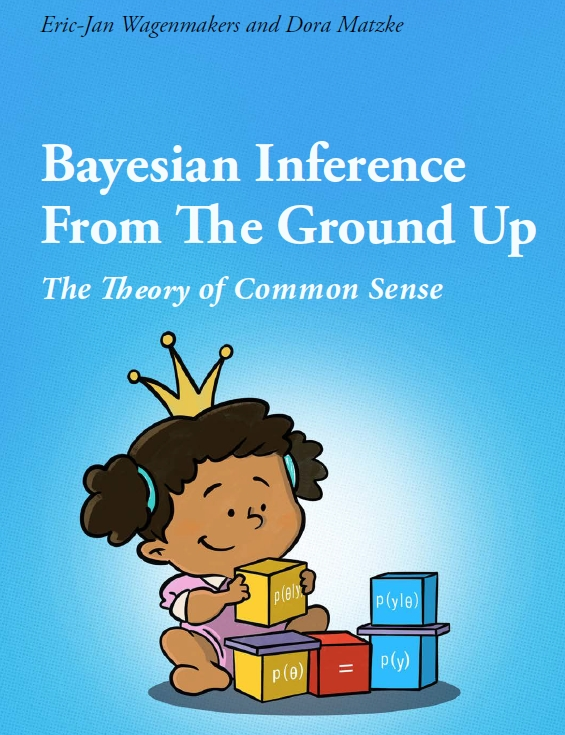# A Free Course Book on Bayesian Inference: [5.] Learning from the Likelihood Ratio

Since 2017, Dora Matzke and I have been teaching the master course “Bayesian Inference for Psychological Science”. Over the years, the syllabus for this course matured into a book (and an accompanying book of answers) titled “Bayesian inference from the ground up: The theory of common sense”. The current plan is to finish the book in the next few months, and share the pdf publicly online. For now, you can click here or on the cover page below to read the first 153 pages. Today we are adding another two chapters: “Learning from the likelihood ratio”, and “An infinite number of hypotheses”. These chapters form the start of part II, “Coherent Learning, Laplace Style”.

In “Learning from the likelihood ratio”, we discuss the scenario of two point hypotheses, which is arguably the simplest inferential scenario: “Miruna comes home and discovers that it’s Dutch pancakes for dinner. Hurray! She knows the pancakes were baked by either of her parents, Andy and Bobbie, but she does not know which one. The only clue as to the identity of the baker is provided by the composition of the pancakes: Andy has a probability of producing a bacon pancake of θA = 0.40, whereas that probability is θB = 0.80 for Bobbie.”

In “An infinite number of hypotheses”, we first increase the number of candidate bakers from 2 to 11; next, we discuss the pancake proclivity of Mr. X, whose value of  θ can take on infinitely many values. It is only here that we introduce the beta distribution. In many introductory texts, the beta distribution is the point of departure. However, the beta distribution and the associated results are not so trivial:

“It might seem, indeed, utterly impossible to calculate out a problem having an
infinite number of hypotheses, but the wonderful resources of the integral
calculus enable this to be done (…) But I may add that though the integral
calculus is employed as a means of summing infinitely numerous results, we in
no way abandon the principles of combinations already treated.” (Jevons, 1874)References

Wagenmakers, E.-J., & Matzke, D. (in preparation). Bayesian inference from the ground up: The theory of common sense.

Wagenmakers, E.-J., & Matzke, D. (in preparation). Bayesian inference from the ground up: Common sense in practice.